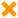# Multiply

 Block Group: Math Operations Icon:The Multiply block multiplies its arguments.

For information on using dataflow blocks, see Dataflow.

For accepted and excluded arguments of Math Operations blocks, see Math Operations.

## Input/Output Property

The following property of the Multiply block can take input and give output.

• input n (number)

input n specifies one of the numbers to multiply.

## Output Property

The following property of the Multiply block can give output but cannot take input.

• output (number)

output returns the result of multiplying all of the input values.

## Examples

The following image shows two examples of the Multiply block. In the rightmost Multiply block, two different data types are used: a number and a string representation of a number.